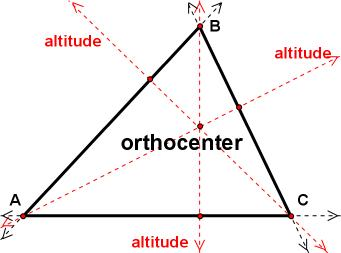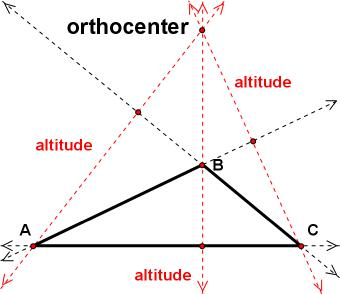index: click on a letter A B C D E F G H I J K L M N O P Q R S T U V W X Y Z A to Z index index: subject areas numbers & symbols sets, logic, proofs geometry algebra trigonometry advanced algebra & pre-calculus calculus advanced topics probability & statistics real world applications multimedia entrieswww.mathwords.com about mathwords website feedback

 Orthocenter The point at which the three (possibly extended) altitudes of a triangle intersect. The orthocenter is one of the centers of a triangle. Orthocenter of an Acute Triangle (Click on the image or here to launch an interactive java applet)Orthocenter of an Obtuse Triangle